Select Page

“Thou shalt not divide by zero.”

So goes one of the great commandments of mathematics classrooms, a rule that must never be broken; otherwise, computers will crash and explode, black holes will spontaneously form, and the universe as we know it will come to an abrupt end.

But rules are meant to be broken.

What if I told you that, despite what you may have learned in school, you can divide by zero if you just think of it in the right way? And what if the answer you get not only had real-world significance, but could actually explain why other parts of math work the way they do? If you’re not afraid to question what you’ve been told, and you’re willing to be flexible with math, then read onward to discover…

# How to Divide by Zero

### Bill Shillito

Note:  If you are using the Chrome Extension “TeX All the Things”, you need to turn it OFF or most of the equations on this page will not render!

Here’s a video of a related talk that I gave in November 2019 at University of Missouri – Kansas City.

### 1. Math = apples, apparently

If we’re going to attempt to figure out how we can divide by zero, we need to first get an idea of why we can’t … or so they say.

To begin with, when you first learn about division, it’s usually in the context of sharing. Say, you’ve got ten apples (because for some reason it’s always apples or cookies or something) and you want to share them equally between two people. Then each person would end up with five apples — in other words, $$10\div 2=5$$.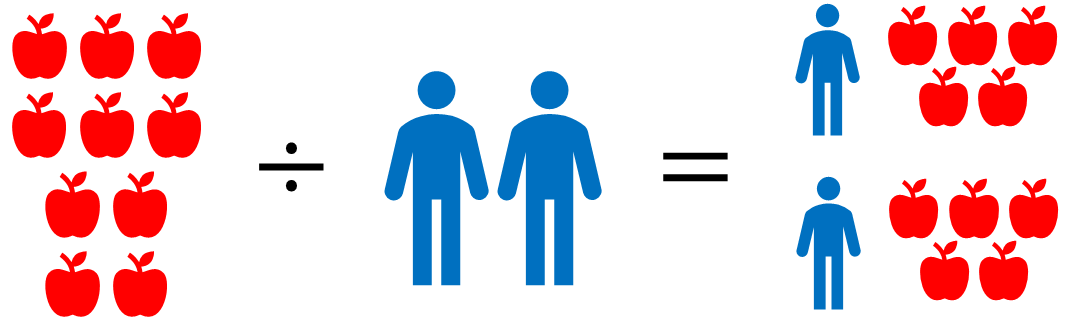But what if we take those ten apples, and we divide them among zero people — what happens then? What would $$10\div 0$$ be based on this situation? The answer isn’t so clear. Maybe it’s zero, because nobody’s getting any apples. Maybe it’s ten, because the apples aren’t being divided at all. Maybe it just doesn’t even make any sense! Since nobody can agree on a logical answer, we end up just leaving division by zero undefined.

But is this really a good enough reason to decide that it’s impossible?

Say we tried to visualize $$10\div\frac{1}{2}$$. How are we going to divide those ten apples between half a person?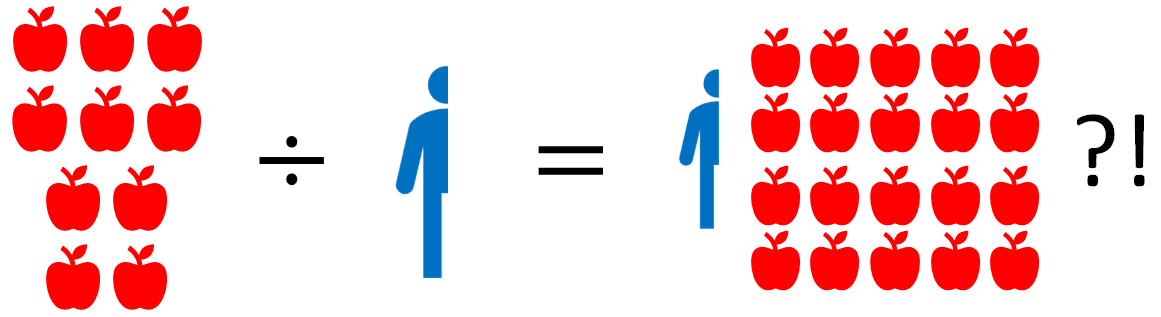This question doesn’t make any kind of real-world sense either, but by that “flip-and-multiply” rule you probably had shoved down your throat for working with fractions, $$10\div\frac{1}{2}$$ is supposed to equal $$20$$. How is that possible? It’s not the mathematical expression that’s invalid, but the situation we’re trying to use to describe it.

Could it be that there’s a context where division by zero makes logical sense, but we just haven’t found it yet?

Well, there is another way we can interpret division in the real world. Suppose the number we’re dividing by isn’t the number of groups, but the size of each group. Then if we ask what $$10\div 2$$ is, we’re basically asking, given ten apples, how many times can we give away two at a time before we run out? The answer is five times.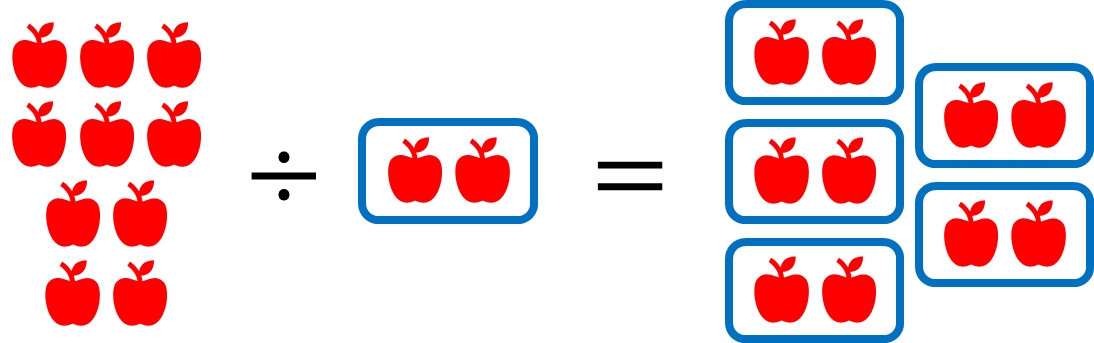Now $$10\div\frac{1}{2}$$ makes sense as well, because we can give away half an apple twenty times.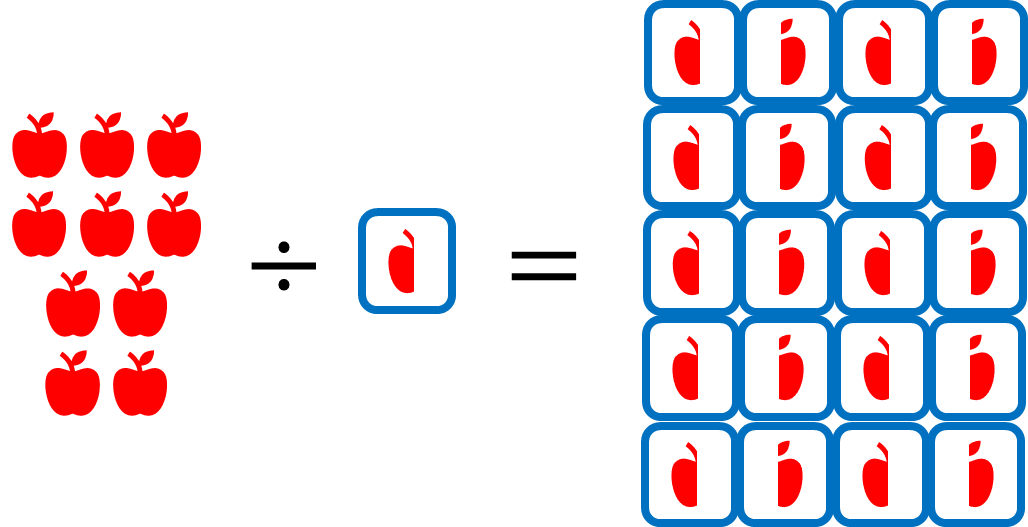So what about $$10\div 0$$? How many times could we give away zero apples? Well … as many times as we want! If we never give anything away, we’ll never run out! So would that mean the answer is …

infinity?

Nah, that’s too weird…

### 2. Less fruit, more math

Let’s try another approach. Early on, you learn that any sentence about division can be rearranged into an equivalent sentence about multiplication. So when we say $$10\div 2=5$$, that also means $$5\times 2=10$$.

But what about division by zero? If we say that $$10\div 0$$ is some number, then that number times $$0$$ would have to be $$10$$. That’s impossible, because anything times zero has to be zero — so again, division by zero is undefined, and the situation looks pretty bleak.

Now, one thing mathematicians often do when they get stuck is look for a pattern and see what it can reveal. Maybe instead of dividing by zero directly, we can just divide by smaller and smaller numbers that approach zero! This idea is what we would call a limit in math. So let’s try dividing $$1$$ by increasingly smaller numbers.

\begin{align*} 1\div\frac{1}{10} &= 10 \\ 1\div\frac{1}{100} &= 100 \\ 1\div\frac{1}{1,\!000,\!000} &= 1,\!000,\!000\\ &\vdots \end{align*}

As the number we divide by gets smaller and smaller, our answer gets larger and larger. But that means … those answers are approaching infinity again? Maybe we’re onto something with this infinity thing! All our evidence so far seems to suggest that $$1\div 0=\infty$$.

There’s just one problem. We’ve forgotten about something important:

Negative numbers.

If we approach zero from the other side, dividing by smaller and smaller negative numbers, our answer still gets larger and larger, but in the negative direction:

\begin{align*} 1\div\frac{-1}{10} &= -10 \\ 1\div\frac{-1}{100} &= -100 \\ 1\div\frac{-1}{1,\!000,\!000} &= -1,\!000,\!000\\ &\vdots \end{align*}

So now our answer is instead approaching negative infinity. That’s a problem — how do we decide which is correct?  Is it $$+\infty$$ or $$-\infty$$?

It seems that we’re at another impasse, but we’ve actually made some progress. We went into this thinking division by zero was impossible … now, looking at limits, we’ve got two possible answers. And they’re both some sort of infinity.

Graph of $$y=\displaystyle\frac{1}{x}$$.  Move your mouse or drag either the red or black point.
Notice that as $$x$$ approaches zero, $$\displaystyle\frac{1}{x}$$ blows up to $$+\infty$$ or $$-\infty$$.

Now I know what you may be thinking — “But infinity isn’t a number! It’s a concept!”

The thing is, our concept of what is or isn’t a “number” has changed throughout history. It took a while for humans to come up with the idea of zero as a placeholder, and even then it wasn’t considered to truly be a number until the fifth century in India. Much of the world used to consider negative numbers to be “absurd” and meaningless for most of recorded history, and today we still call square roots of negative numbers “imaginary” — a label originally intended to dismiss them as nonsense — even though they’re essential in describing the real, physical world!  (Don’t believe me?  Ask an electrical engineer.)

Every time we expand our definition of “number”, we deepen our understanding of mathematics. Infinity is no different — plenty of serious mathematicians use infinity as a number all the time without any problems. One way they do so is by referring to the extended real number line — just like the regular real number line, but with two extra points added at the ends to correspond with $$+\infty$$ and $$-\infty$$. The extended real number system follows many of the same rules as the usual real numbers, but with some extra rules to explain how infinity works.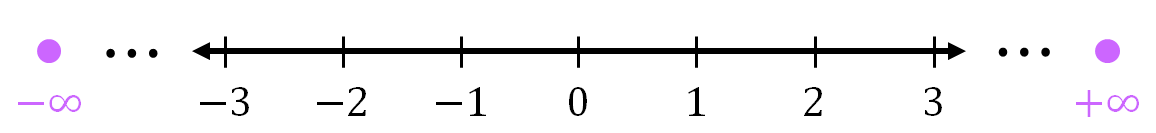##### READ MORE: Properties of the extended real numbers

Most of the rules followed by the extended real numbers are exactly what you’d expect, as long as you’re careful to avoid certain indeterminate expressions.  (We’ll often use $$\infty$$ to mean $$+\infty$$.)

• $$+\infty$$ and $$-\infty$$ absorb most numbers by addition and subtraction.
\begin{align*} \infty+1&=\infty\\ 2+\infty&=\infty\\ 4-\infty&=-\infty\\ \infty+\infty&=\infty \end{align*}
• $$+\infty$$ and $$-\infty$$ also absorb most numbers by multiplication and division, while obeying the usual rules for multiplying positive and negative numbers.
\begin{align*} 5\cdot\infty&=\infty\\ \infty\cdot -3&=-\infty\\ -\infty\cdot-\infty&=\infty\\ \frac{\infty}{-1.3}&=-\infty \end{align*}
• Dividing any finite number by $$+\infty$$ or $$-\infty$$ gives zero.
\begin{align*} \frac{3}{\infty}&=0\\ \frac{\pi}{-\infty}&=0 \end{align*}
• Indeterminate expressions involving $$+\infty$$ and $$-\infty$$ are illegal.
\begin{align*} \infty-\infty&=\text{Illegal!}\\ 0\cdot\infty&=\text{Illegal!}\\ \frac{\infty}{\infty}&=\text{Illegal!} \end{align*}

Computers also make use of positive and negative infinity as part of floating-point arithmetic, which is a system for representing numbers of different magnitudes, including infinity, as accurately and efficiently as possible.

In fact, floating point arithmetic actually has a way of dealing with division by zero, though it cheats a little — it actually splits zero into two different numbers: positive zero and negative zero. Using these signed zeros, as they’re called, we have $$1\div(+0)=+\infty$$ and $$1\div(-0)=-\infty$$ … much like the limits we saw earlier!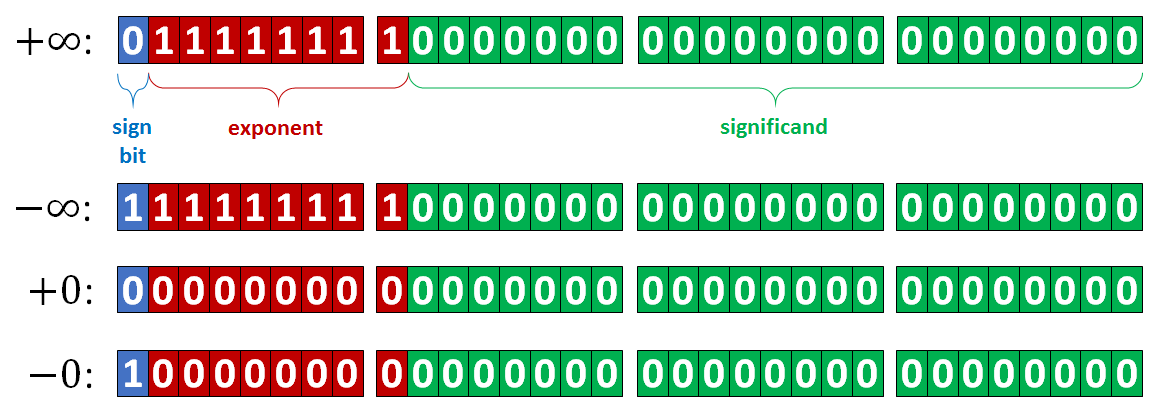This is a bit of a hack though, since in the real number system, zero is neither positive nor negative — it’s unsigned, sitting on the boundary but taking neither side. Positive zero and negative zero are both really just zero.

So as far as division by zero is concerned we’re still stuck between two answers, infinitely far apart from each other, with seemingly no hope of reconciling them — unless positive infinity were equal to negative infinity or something weird like that.

Hah … like that would ever happen.

### 4. A new view of infinity

Sometimes, to make progress on a mathematical problem, you need a change in perspective. Literally.

Around the time of the Renaissance, artists wanted to develop a mathematical foundation for perspective so that their art could be made to look more realistic. These studies, along with the results of certain ancient Greek mathematicians before them, became the basis for what we know nowadays as projective geometry.

One key idea of projective geometry is that when you represent parallel lines in perspective, they actually converge to a single point on the horizon. Mathematicians call this a point at infinity; artists call it a vanishing point.Those white lines are parallel. I promise.

Let’s see if we can use some of the ideas from projective geometry to better understand infinity. In order to take a look at the real number line in perspective, we’ll need to be working in two dimensions instead of one, so we’ll imagine the number line as just the horizontal axis in the plane. Then we’ll draw a circle of radius $$1$$, centered at the origin.  (You can see an interactive version of this construction below.)

What we’re going to attempt to do is match points on the number line to points on this unit circle, so that every point on one pairs up perfectly with a point on the other. We can fix one point at the top of the circle, and then draw a line that connects it to some point on the number line, kind of like a ray of light. That line is also guaranteed to intersect that circle at some second point — the stereographic projection of that real point onto the circle. We can even use a little bit of algebra (see the drop-down below) to show exactly where that projection is.

The big idea here is that every real number now has a “home” somewhere on that circle. All the positive numbers live on the right, and the negative numbers live on the left. Zero is at the very bottom, and at the far right and left sides live $$1$$ and $$-1$$.

Move your mouse or drag the blue point to see how $$P(a)$$ changes as $$a$$ changes.

##### READ MORE: Projecting the real line onto the unit circle

Let $$a$$ be a real number, which we can associate with the point $$(a,0)$$ on the real axis. If we fix the point $$(0,1)$$, then the line through these two points has equation $$y=\frac{-1}{a}x+1$$. We want to find where this point intersects the unit circle, whose equation is $$x^2+y^2=1$$.

We can substitute the first equation into the second and begin to solve for $$x$$:

\begin{align*} x^2+\left(\frac{-1}{a}x+1\right)^2&=1\\ x^2+\frac{x^2}{a^2}-\frac{2}{a}x+1&=1\\ x^2\left(1+\frac{1}{a^2}\right)-\frac{2}{a}x&=0\\ x\left[x\left(1+\frac{1}{a^2}\right)-\frac{2}{a}\right]&=0\\ \end{align*}

We have two possibilities now — either $$x=0$$, or $$x\left(1+\frac{1}{a^2}\right)-\frac{2}{a}=0$$.  The former solution is just the point at the top of the circle, while the second one is the one we want.

\begin{align*} x\left(1+\frac{1}{a^2}\right)-\frac{2}{a}&=0\\ x\left(1+\frac{1}{a^2}\right)&=\frac{2}{a}\\ x&=\frac{\frac{2}{a}}{1+\frac{1}{a^2}}\cdot\frac{a^2}{a^2}\\ x&=\frac{2a}{a^2+1}\\ \end{align*}

Now that we have $$x$$, we can substitute this back into the first equation to find $$y$$:

\begin{align*} y&=\frac{-1}{a}\left(\frac{2a}{a^2+1}\right)+1\\ y&=\frac{-2a}{a(a^2+1)}+1\\ y&=\frac{-2}{a^2+1}+\frac{a^2+1}{a^2+1}\\ y&=\frac{a^2-1}{a^2+1} \end{align*}

Thus for any real number $$a$$, its projection onto the unit circle is $$P(a)=\left(\displaystyle\frac{2a}{a^2+1},\displaystyle\frac{a^2-1}{a^2+1}\right)$$.

If you think these formulas seem strangely like double-angle identities with the tangent function, you’re not too far off!  If we make the substitution $$a=\tan(\frac{1}{2}\theta)$$, where $$-\frac{\tau}{2}<\theta<\frac{\tau}{2}$$*, then the formula reduces to $$P(a)=(\sin{\theta},-\cos{\theta})$$.  This shows that $$\theta$$ is the angle from $$P(0)$$ to $$P(a)$$.

* Note:  $$\tau$$ denotes the circle constant $$6.283185…$$, also equal to $$2\pi$$.

So what lives at the top of the circle? If we sweep out to the right, we can see that larger and larger numbers live closer and closer to the point at the top. So, we might imagine that at the very top of the circle lives infinity. Also, notice that the line coming from the top of the circle is parallel to the horizontal axis — and if we were to look at this in perspective, these two lines do indeed intersect all the way out at infinity.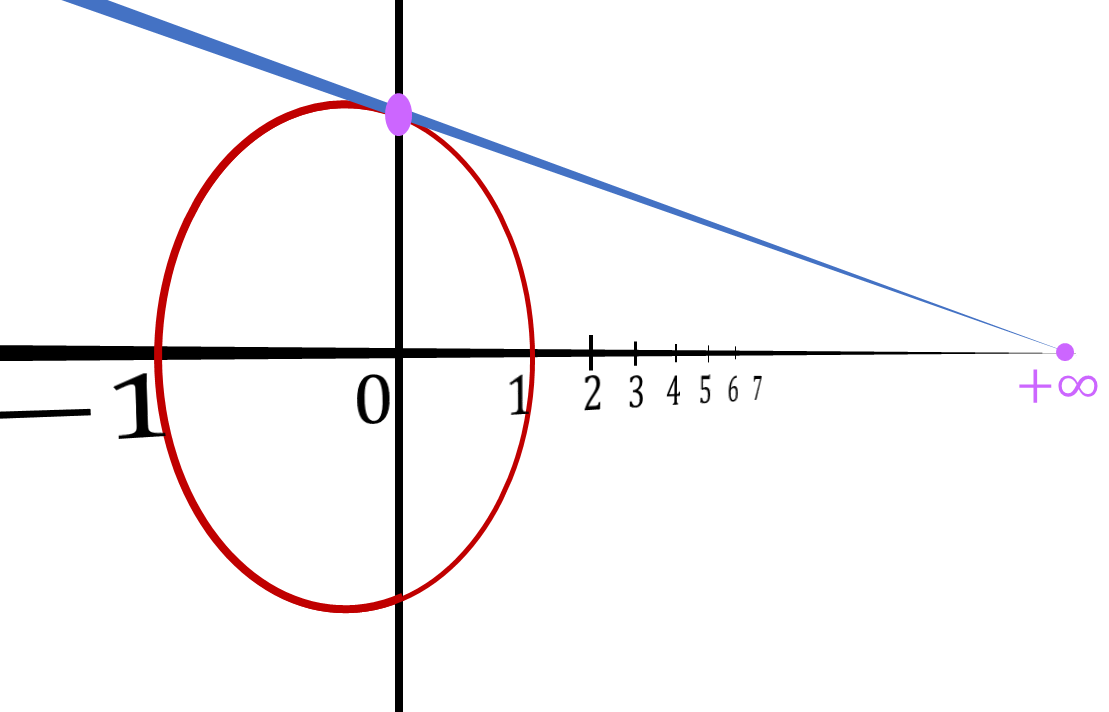But what if we sweep out to the left? As we look at larger and larger negative numbers, they end up living closer and closer to that same point at the top, but they approach from the other side. And if we go all the way, and look at the negative part of the number line in perspective, then we’ve found that again the lines intersect at negative infinity. So, somehow, negative infinity also lives at that same point on the top of the circle.

We’ve managed to show that, given the right perspective, positive and negative infinity are actually connected to each other, and in some sense can be viewed as the same point, the same number — another boundary between the positive and negative numbers, just like zero! In some sense, we’ve discovered a new number!

Let’s give it a name.

Like zero, this new number seems to be unsigned — neither positive nor negative — so let’s call it unsigned infinity, and let’s write it with a tilde (a little squiggle, like in the Spanish “ñ”) on top.

## ${\Huge\overset\sim\infty}$

This new structure, where we’ve added a single infinity to the real numbers instead of two, has its own special name — the projectively extended real number line. It’s another valid number system that contains all of the real numbers, but has its own set of special rules for how to deal with our new kind of infinity.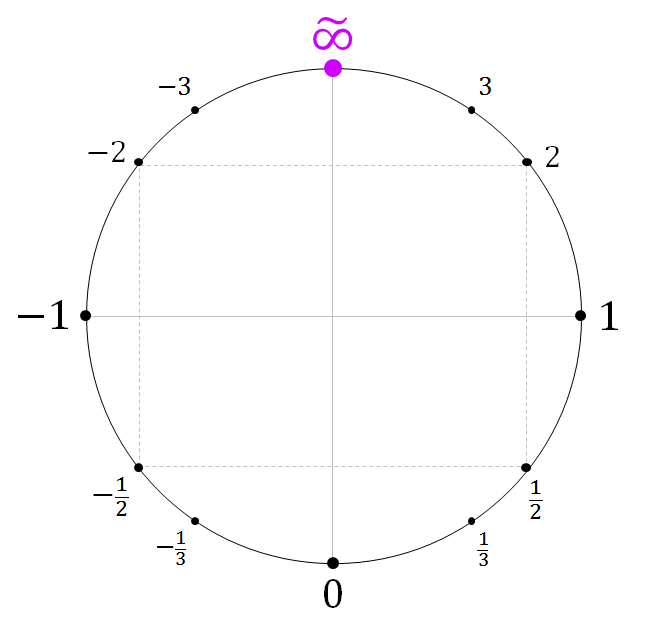### 5. In which we literally actually divide by zero

So what else can we uncover about this new number system? After all, circles have a whole lot of symmetry, so we’re bound to find at least something interesting.

Well, based on the way we set up our projection, it should be no surprise that for every positive number on the right, its corresponding negative — its opposite — lives at the opposite point on the left, a perfect reflection over the vertical axis.

But there’s an even more interesting kind of symmetry hidden here as well. See how $$\frac{1}{2}$$ lives right under $$2$$? This is actually true in general: given some number, the reciprocal of that number — that is, the result of dividing $$1$$ by that number, basically its upside-down cousin — is also given by a reflection, but this time over the horizontal axis. We can even prove it with a little more algebra.

##### READ MORE: Proving the reflections

Again we’ll use the fact that $$P(a)=(x,y)=\left(\displaystyle\frac{2a}{a^2+1},\displaystyle\frac{a^2-1}{a^2+1}\right)$$.

First let’s find $$P(-a)$$:

\begin{align*} P(-a)&=\left(\frac{2(-a)}{(-a)^2+1},\frac{(-a)^2-1}{(-a)^2+1}\right)\\ &=\left(\frac{-2a}{a^2+1},\frac{a^2-1}{a^2+1}\right)\\ \end{align*}

Note that the $$x$$-coordinate has flipped sign, while the $$y$$-coordinate is the same.  Thus $$P(-a)=(-x,y)$$.

Now let’s find $$P(\frac{1}{a})$$:

\begin{align*} P\left(\frac{1}{a}\right)&=\left(\frac{2\left(\frac{1}{a}\right)}{\left(\frac{1}{a}\right)^2+1},\frac{\left(\frac{1}{a}\right)^2-1}{\left(\frac{1}{a}\right)^2+1}\right)\\ &=\left(\frac{\frac{2}{a}}{\frac{1}{a^2}+1}\cdot\frac{a^2}{a^2},\frac{\frac{1}{a^2}-1}{\frac{1}{a^2}+1}\cdot\frac{a^2}{a^2}\right)\\ &=\left(\frac{2a}{1+a^2},\frac{1-a^2}{1+a^2}\right)\\ \end{align*}

This time, the $$x$$-coordinate is the same, while the $$y$$-coordinate has been flipped.  Thus $$P(\frac{1}{a})=(x,-y)$$.

But wait … if we can divide $$1$$ by any number just by reflecting that number upside down on the circle … then what’s keeping us from doing that to $$0$$?

There’s only one possible inevitable conclusion, staring us right in the face: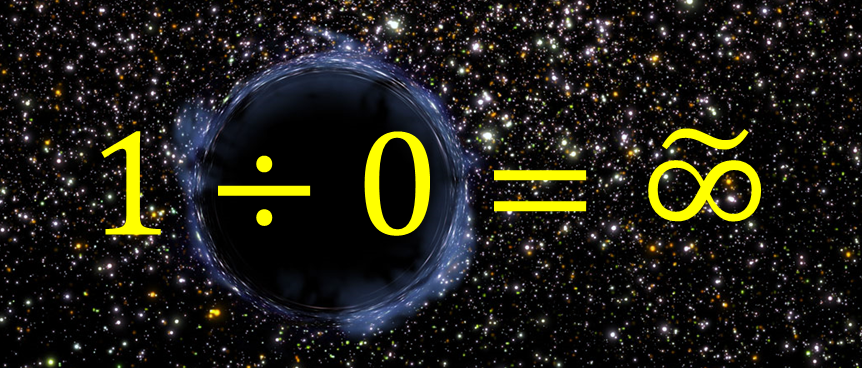There we have it:  one divided by zero equals unsigned infinity. And, by extension, so does almost any other number divided by zero (with the sole exception of $$0\div 0$$, which we’ll talk about later).

Not only does this reconcile our two conflicting answers that we got earlier, but it does so in a beautifully symmetric way, because our relationship goes the other way as well:

${\large 1\div \overset\sim\infty=0}$

We’ve uncovered an underlying duality between zero and infinity, the small and the large, nothing and everything.

Mind = blown.

### 6. Does infinity really exist?

This may all just sound like mere abstract nonsense — how could positive and negative infinity possibly be connected except in some weird theoretical sense that has absolutely no connection to the real world?

But you can actually see this connection for yourself using nothing but a spoon — or any other concave mirror, the kind that’s sometimes called a “magnifying mirror” in department stores.

If you bring an object close to a concave mirror, its reflection is right side up. Then, as you move it away, the mirror image gets larger and larger, until for a split second it fills the entire mirror, and then suddenly the image is upside-down and shrinking again. If we use some basic equations from optics to figure out the magnification at this focal point in the middle, we find that physics itself is dividing by zero, and as a result, the mirror image is infinitely large and sitting at the boundary between right side up (positive magnification) and upside down (negative magnification).

Drag the object to see what happens to the image.
The dotted green and purple lines converge at the tip of the image.
When those lines are parallel, the image is at infinity.

Variables:

• $$f=$$ focal length of mirror
• $$d_o=$$ distance of object from mirror
(assumed to be positive)
• $$h_o=$$ height of object
(positive = right side up)
• $$d_i=$$ distance of image from mirror
(positive = in front of mirror, negative = behind mirror)
• $$h_i=$$ height of image
(positive = right side up, negative = upside down)
• $$m=$$ magnification
(positive = right side up, negative = upside down)

Mirror/lens equation:

$\frac{1}{f}=\frac{1}{d_i}+\frac{1}{d_o}$

Magnification equation:

$m=-\frac{d_i}{d_o}=\frac{h_i}{h_o}=\frac{f}{f-d_o}$

If object is at focus, then $$d_o=f$$, so:

$m=\frac{f}{0}=\overset\sim\infty$

We’ve just seen a manifestation of division by zero and unsigned infinity in the real world.

Mind = double-blown.

### 7. Everything you never knew you could do with infinity

There’s plenty of new doors that are now unlocked by connecting the two infinities and allowing division by zero — doors that help explain why the rest of mathematics works the way it does.

For example, we used to say that the slope of a vertical line was undefined; now we can just say that it’s infinity (unsigned of course), and since horizontal lines have a slope of zero, perpendicular lines have opposite reciprocal slopes just like we’d expect.

Going to trigonometry, this also means the tangent of a right angle also ends up being infinity for the same reason. And if we want the cotangent of a right angle — which lots of beginning trig students mistakenly think is undefined — we can easily find it using infinity:

$\cot(90^\circ) = \frac{1}{\tan(90^\circ)} = \frac{1}{\overset\sim\infty} = 0$

Going off on that “tangent” (forgive the pun), graphs can not only “cross through” or “bounce off” zero, but also infinity, leading to a much more symmetrical way to understand the behavior of polynomial, rational, and trigonometric functions.We can even apply this idea to conic sections, letting an ellipse send off one of its foci to infinity, becoming a parabola for a split second, and then coming out the other side, twisted in the process, to reveal a hyperbola.

So much of math reveals bizarre and beautiful new worlds when we question our underlying assumptions, dare to dream a little, and explore new avenues of thought.

### 8. Complexity is beautiful

The relationships only become even more profound when we repeat our construction in the complex numbers — that is, all the numbers of the form $$a+bi$$, where $$a$$ and $$b$$ are real and $$i$$ is the square root of $$-1$$.

Remember that the complex numbers are best understood not as a line of numbers, but as a plane of numbers. We can repeat our construction by adding a third dimension (so that we have somewhere to project from), using the unit sphere instead of the unit circle, placing a point at the top of the sphere and drawing the line connecting it to any point we’d like on the complex plane. That line again intersects the sphere at exactly one other point for any finite complex number, so we think of that point as where the complex number “lives” on that sphere.

When we go off to infinity in any direction, though, the projection always ends up at the top of the sphere! Again we label this point at the top as $$\overset\sim\infty$$, but since the complex numbers have not just positives and negatives but infinitely many directions, we call it complex infinityThe sphere we get by including complex infinity is often called the Riemann sphere, and it, too, allows us to divide by zero. The Riemann sphere is a fundamental object in branches of mathematics like complex analysis and algebraic geometry — truly beautiful subjects that really tie together the rest of mathematics.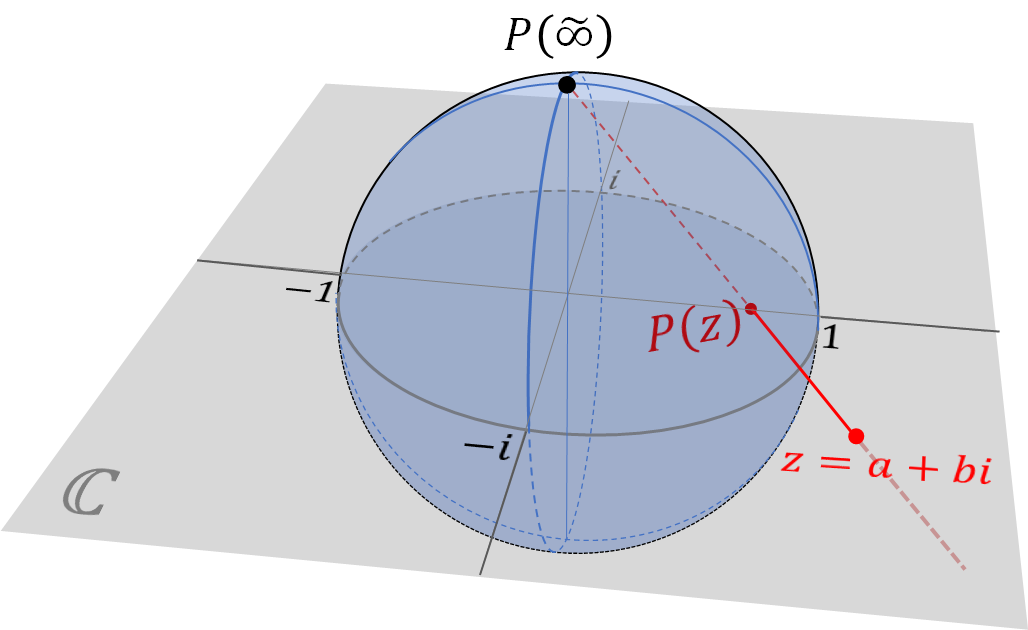### 9. Fielding an objection

It may seem like we’ve made some kind of universe-shattering discovery that will forever change the face of mathematics as we know it. But surprisingly, it actually isn’t all that new of an idea — plenty of mathematicians already know about the projective reals and use them all the time.

So why don’t all the textbooks teach it this way? Why don’t we learn from the get-go that division by zero yields this special infinity? Don’t students all over the world deserve to know the truth?

To get some idea of why this isn’t being shouted from the rooftops, we need to look a little more closely at the algebraic rules that govern our new number system we’ve created.

A very important concept in the study of algebra is the idea of a field. For our purposes, we can think a field of as a number system in which the four classical operations of addition, subtraction, multiplication, and division work “nicely”, meaning that they follow particular rules that make computations easy, especially when it comes to algebra. We can rearrange and regroup terms, and even cancel them out using inverses — basically everything you’d need to solve an algebraic equation. The sole exception is that zero doesn’t have a multiplicative inverse, since it can be proven — not just assumed! — that in any field, anything times zero equals zero, never one.

In abstract algebra, a field is a set of numbers (or number-like objects) containing two operations, called addition ($$+$$) and multiplication ($$\times$$), which follow certain rules.

Addition and multiplication are commutative, meaning that the order of numbers doesn’t matter:

$a+b=b+a\qquad a\times b=b\times a$

Addition and multiplication are also associative, meaning that we can use parentheses to group numbers however we want:

$(a+b)+c=a+(b+c)\qquad (a\times b)\times c=a\times (b\times c)$

Both addition and multiplication have a (unique) identity element, which leaves any other number alone:

$a+0=a\qquad a\times 1=a$

Almost every number has an inverse under both addition and multiplication (except zero in the latter case), so that combining a number with its inverse yields the identity:

$a+(-a)=0\qquad a\times\frac{1}{a}=1$

This property is what basically allows us to define subtraction and division in terms of addition and multiplication.

Finally, the relationship between addition and multiplication is given by the distributive property:

$a\times(b+c)=(a\times b)+(a\times c)$

These properties are what allow us to use algebra to solve equations.  Any time you subtract a term from both sides of an equation like $$3x+1=10$$, you’re making use of the associative, inverse, and identity properties to simplify the equation and isolate $$x$$.

But wait … we just found that $$1\div 0=\overset\sim\infty$$… wouldn’t that mean that $$0\cdot\overset\sim\infty=1$$?

That seems plausible, but then remember that $$2\div 0=\overset\sim\infty$$ as well, so then we’d have $$0\cdot\overset\sim\infty=2$$.

But then … $$1=2$$?

What the heck? Something is wrong here.

Well, most people would say that our problem was dividing by zero in the first place. But let’s look a bit closer and see if we can figure out where the true problem is.

### 10. The absorbing power of numbers

(From here on by the way, when we write “infinity” or “$$\infty$$”, you can interpret it as positive, negative, or unsigned, depending on which context is most appropriate.)

An interesting thing about both zero and infinity is that they have a tendency to “absorb” other numbers — zero absorbs numbers by multiplication, but so does infinity. When these two forces compete, the results are catastrophic: we end up with contradictions like we saw before.

Zero times infinity is what we call an indeterminate expression — an expression that, if you play with it enough, you could get to equal any number: $$1$$, $$2$$, $$-37\frac{1}{2}$$, $$0$$, even $$\infty$$.

For instance, suppose we did assume that $$0\cdot\infty=1$$. Then, we could exploit the absorbing properties of zero to show that $$1=2$$. And if we can’t tell the difference between one and two, or for that matter any two numbers, then all of arithmetic becomes useless.

\begin{align*} 1&=0\cdot\infty\\ &=(2\cdot 0)\cdot\infty\\ &=2\cdot(0\cdot\infty)\\ &=2\cdot 1\\ &=2 \end{align*}

Oops.

So that’s where the true problem is — while zero and infinity are certainly reciprocals of each other, they’re not truly inverses because they don’t multiply to be one. It isn’t division by zero or the introduction of infinity that causes algebra to break, but the way that zero and infinity interact with each other if we’re not careful.

There are a handful of other indeterminate expressions as well:

$\frac{0}{0}\qquad\frac{\infty}{\infty}\qquad\infty-\infty$

Algebra just isn’t powerful enough to handle these expressions. To do so, we would need to bring out the big guns: calculus.

So this is why when mathematicians were deciding on the proper definition of a field, they decided to keep things simple and sweep the problem under the rug entirely by disallowing division by zero and insisting that infinity isn’t a number.

But it doesn’t have to be this way.

While the projectively extended real number system isn’t a field, it still contains the field of real numbers, and those real numbers still have all the same nice properties. We just have to remember that while infinity is still a number, it’s not a real number, and it’s not part of any field. It follows its own unique rules, and we have to treat it with care, avoiding indeterminate expressions like zero times infinity and a small handful of others.

Now perhaps you’re wondering, “but wait — if we found a good answer to $$1\div 0$$, what’s to stop us from finding some other brand-new number as the answer to $$0\cdot\overset\sim\infty$$ or $$0\div 0$$ and seeing what happens from there?”

If so, congratulations — you’re starting to think like a mathematician! It turns out that there is something called wheel theory in which you can do exactly that … but that’s a whole rabbit hole of its own.

Wheel theory is a relatively new branch of mathematics.  The first paper that rigorously laid out the theory was published by Swedish mathematician Jesper Carlström in 2004.

A wheel is an algebraic structure in which given any two numbers $$a$$ and $$b$$, we can always calculate $$a\div b$$ — even when one or both are zero.  In a wheel, $$1\div 0=\infty$$ (where $$\infty$$ is unsigned) just like we’ve seen, but now $$0\div 0$$ is defined as new element which we’ll call nullity* and denote $$\Phi$$.  In addition, $$0\cdot\infty=\frac{\infty}{\infty}=\Phi$$.  The name “wheel” comes the visual image of the real projective line with $$\Phi$$ added as a point in the center.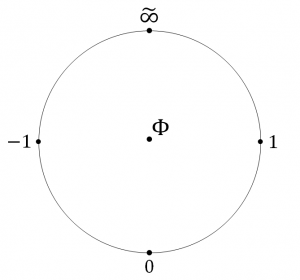The rules of working with $$\Phi$$ are simple:  combining any number with $$\Phi$$ by addition, subtraction, multiplication, or division yields $$\Phi$$.  In some sense, $$\Phi$$ is the ultimate absorptive element.

While it may seem that the introduction of $$\Phi$$ has solved all of our problems with arithmetic, it comes at great cost: many operations that were once simple now become much more complicated.  For example, in a field, $$x-x=0$$ for any number $$x$$; in a wheel, this is not always true (since $$x$$ could be $$\infty$$ or $$\Phi$$).  Instead we must work with the more cumbersome expression $$x-x=0x^2$$; if it happens to be true that $$0x=0$$, then $$x-x=0$$ after all.

It’s worth noting that the $$\Phi$$ from wheel theory is very similar to another construction from floating-point arithmetic:  NaN, which ironically stands for “Not a Number”.  Computers use NaN to signal to programmers that some kind of error has occured as a result of a calculation — like those involving the indeterminate expressions we saw earlier.

More details about wheel theory can be found at the Wikipedia article on the subject.

* Note:  The “nullity” notation and terminology actually comes from English computer scientist James Anderson, who had developed a system called “transreal arithmetic” which came close to wheel theory.  Anderson’s theory unfortunately has some flaws — in particular, he does not unify $$+\infty$$ and $$-\infty$$, and as a result his definitions of $$1\div 0=+\infty$$ and $$-1\div 0=-\infty$$ lead to contradictions — but I prefer his notation to that of Carlström, who calls the $$0\div 0$$ element “bottom” and denotes it $$\bot$$.

### 11. The end is just the beginning

Here’s what I think should be the main takeaway of all this.

Breaking the rules is actually how we often learn more about mathematics — we study what happens in more general situations, so that we can better understand and appreciate the kinds of math we use on a daily basis. From there, we can make the choice ourselves about what number system we want to work with, armed with the knowledge of how to avoid pitfalls and use the numbers available to their full potential.

Mathematics is not set in stone, but an ever-growing and evolving body of knowledge. Many great insights and discoveries have been made just because somebody stopped taking “you can’t do that” as an answer, and instead wondered, “what if you could?”

What assumptions will you question?

What new worlds will you discover?

#### The possibilities are infinite.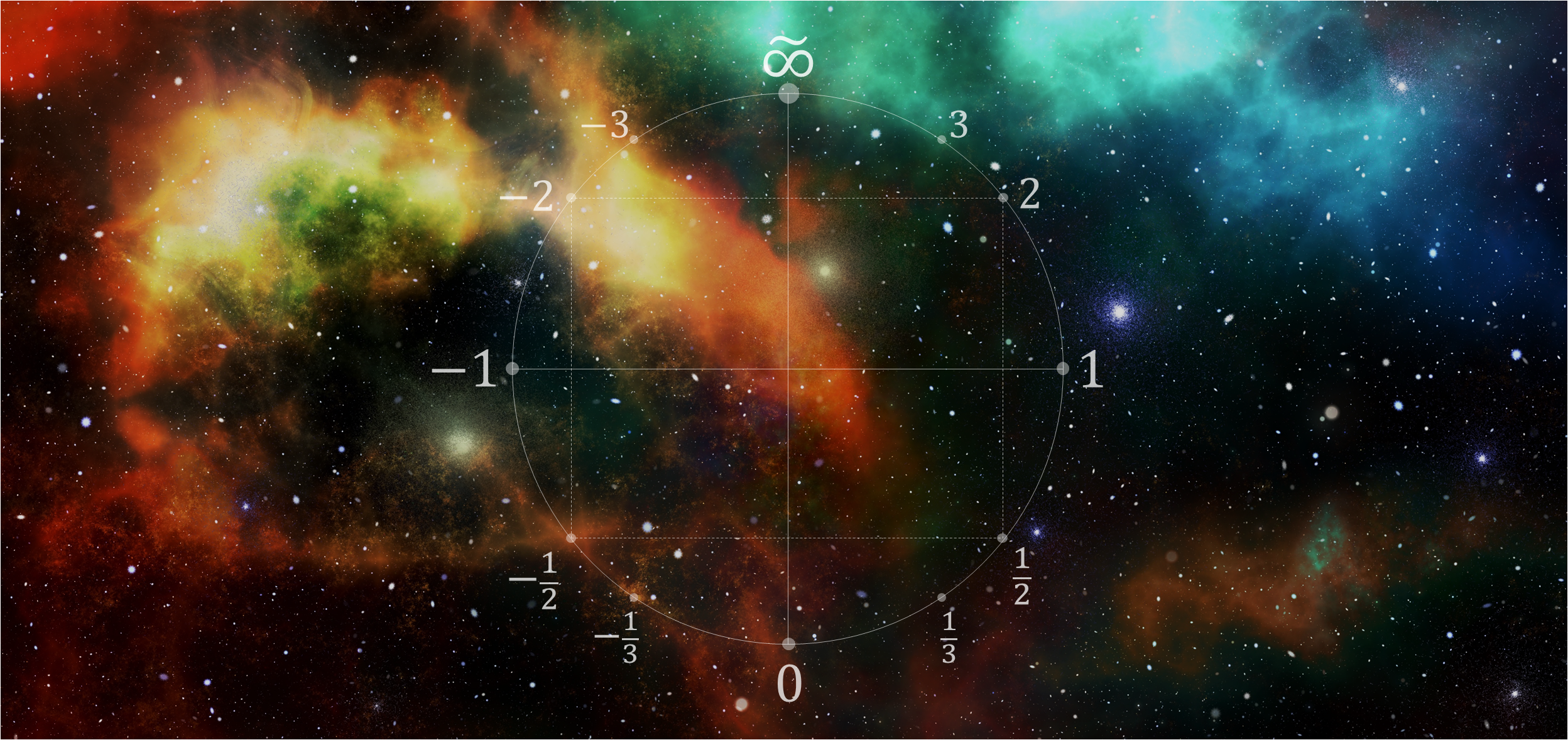## Contact Me

Have questions, comments, or other feedback about what you just read?  Use the form below to shoot me an email!

6 + 3 =